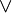Questions day 10:

• What is a multimeter?

“A multimeter measures electrical properties such as AC or DC voltage, current, and resistance. Rather than have separate meters, this device combines a voltemeter, an ammeter, and an hommeter. Electricians and the general public might use it on batteries, components, switches, power sources, and motors to diagnose electrical malfunctions and narrow down their cause.”(http://www.wisegeek.org/what-is-a-multimeter.htm#multimeter-isolated)• What is the difference among BINARY, HEXADECIMAL, and DECIMAL?

“Binary: Binary numbers are just “Base 2” instead of “Base 10”. So you start counting at 0, then 1, then you run out of digits … so you start back at 0 again, but increase the number on the left by 1.

Decimal: To understand Binary and Hexadecimal numbers, it is best to know how Decimal numbers work. Every digit in a decimal number has a “position”, and the decimal point helps us to know which position is which.

Hexadecimal: There are 16 of them. They look the same as the decimal numbers up to 9, but then there are the letters (“A’,”B”,”C”,”D”,”E”,”F”) in place of the decimal numbers 10 to 15. So a single Hexadecimal digit can show 16 different values instead of the normal 10 like this:”

 Decimal: Hexadecimal: 0 1 2 3 4 5 6 7 8 9 10 11 12 13 14 15 0 1 2 3 4 5 6 7 8 9 A B C D E F
• What is the difference between inductive and deductive logic?

“Inductive and inductive reasoning are both approaches that can be used to evaluate inferences. Deductive reasoning involves starting out with a theory or general statement, then moving towards a specific conclusion.Indeductive reasoning on the other hand, takes a series of specific observations and tries to expand them into a more general theory. Each approach is very different, and it is important to be aware that both inductive and deductive reasoning can end up with false results, especially if the initial premise of the reasoning is false, in which case the results are said to be “unsound.””

• What did you think about Honors Academy, Exploratory Math?

I think that was very interesting and fun. I met a lot of different people from other schools and teachers from other countries. I really liked this experice, is my very first time that I’ve been in a summer course. I hope I go to Stanford next year 🙂

Day 9:

We  worked on our blogs and started our research with our partners. I needed to investigate about prisoner’s dilemma.Later we worked on arduino, my group made our arduino sing star wars song with some LEDs that lighted in rythm with the music.

In the the last block we did the posters for our research projcet and finally we worked on our city.

Day 8:

In the morning we worked in the computer lab with new groups. We used the arduinos and did many circuits. Some of them make the LED light in different ways. After break we worked in arduinos again.In the afternoon we reviewed adjacent Matrix and learned Karnaugh’s Map with truth tables.

Then we planned our city “Nyan Ville” with our group 🙂

Questions Day 8!

• Use this website to make
• ~(p ^ q) v (~p v ~q)=• You used 2 chips yesterday, find them and link them to your post. What is the name of the 2 chips that seem most like the ones you used yesterday: http://www.skot9000.com/ttl/

SN54/74LS08 (And Chip)SN54/74LS32

• What is a truth table?

A truth table is a convenient and helpful way to organiza true values of different satements. A truth table is a table whose columns are statements, and whose rows are possible scenarios. The table contains every possible scenario and the truth values that would occur. Every statement is either True or False.

• What is Demorgan’s Law?

¬ is the negation operator (NOT)is the conjunction operator (AND)is the disjunction operator (OR)

⇔ means logically equivalent (If and only if)

• What is Ohm’s Law?

Ohm’s Law is the mathematical relationship among electric current (I), Resistance(R), voltages(V).For many conductors of electricity, the electric current flows through them is directly proportional to the voltage applied to them.

• What is double implication?(⇔)means that P and Q are equivalent. So the double implication is true if P and Q are both true or if P and Q are both false; otherwise, the double implication is false” (http://www.millersville.edu/~bikenaga/math-proof/truth-tables/truth-tables.html)

• What is an adjacency matrix?

“An adjacency matrix is a matrix which describes a graph by representing which vertices are adjacent to which other vertices.” (http://en.wikipedia.org/wiki/Adjacency_matrix)